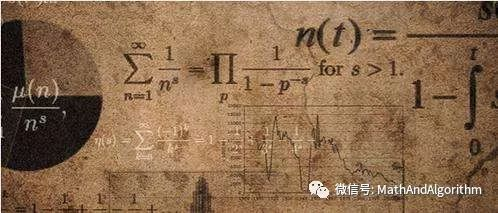# The rise of pure mathematics

• Time:
• From:AIAT
• Author:atr
• Views:225The invention of calculus

calculus has brought many successful applications, but its shortcomings have also attracted many criticisms. Some mathematicians' handling of these problems in the nineteenth Century led to the emergence and development of pure mathematics. Today, pure mathematics has become the mainstream of mathematics.

> > > >

(1) physical research is the objective material world, the mathematical research is the concept of the world that people want to come out.

(2) the truth of physics is tested by observation and experiment. It has some limitations, but the truth of mathematics has the property of "absoluteness". A simple example: PI after the decimal point 100th, 1000th and any one is only in the physical and digital; universal constant light speed or Planck constant, no matter what unit, needless to say 100 unreliable, fiftieth bits and thirtieth bits are also can accurately achieve it's hard to say.

("font-size: 3) in terms of the method, mathematics on head, and the physics experiment, because of this, physics after Newton into three branches: Theoretical Physics, experimental physics, mathematics and physics. Only in extremely individual cases, mathematics has been mentioned in theoretical and Experimental Mathematics (a lot of people have done the test of coin throwing). But in the natural sciences, almost no one talks about pure physics, pure chemistry, but a mathematical counterpart - pure mathematics.

The appearance of pure mathematics in

pure mathematics word appeared officially in the mathematical literature is at the beginning of nineteenth Century, there were three major mathematics journals officially marked with pure mathematics words, they are: 1810, the French mathematician Ge M Gore Na (J. D. Gergonne, 1771 - 1859) of pure and Applied Mathematics founded "(Annales de math matiques pures et appliqu es); German mathematician Kyle (1826 A. L. Crelle, 1780 - 1855) of pure and Applied Mathematics Journal" (Journal f die Reine und Angewandte Mathematik R), often referred to as the "Kyle" magazine in 1836; the French mathematician Liu Weier (J. Liouville, 1809 - 1882 pure and Applied Mathematics) magazine, and Kyle magazine "competition" (Journal de math matiques pures et appliqu es).

the three mathematics journals invariably choose the "pure mathematics" title that: the concept of pure mathematics has matured; pure mathematics is the antithesis of Applied Mathematics; pure mathematics has certain legal status, for its expanding foundation.

Please note that these are the whole society revolutionary situation inseparable, specific to mathematics, mathematicians began occupation and specialization, they not only to teaching, but also engage in scientific research, engage in scientific research should have the professional magazine published results, no results alone promoted promotions, even your job would. For 200 years, the banner of pure mathematics has provided an opportunity for many talented mathematicians to innovate. In this sense, the Norwegian Abel (N. H.Abel, 1802 - 1829) can be said to be the first pure mathematician . Today, pure mathematics has become the mainstream of the whole mathematics.

from elementary mathematics to higher mathematics

calculus was invented in the late seventeenth Century by British scientist Newton (I. Newton 1643, 1727) and German scientist Leibniz (G. W. Leibniz, 1646 1716). We are used to call the former mathematics elementary mathematics, and regard calculus and its later development, especially differential equations (including ordinary differential equations and equations, partial differential equations and equations, variational methods, etc.) as advanced mathematics. In order to keep the calendar

From: 纯粹数学的兴起
Translate by: Baidu Translate .